### Extended Math [v1.0]

#### MrD69

Stencyl Extended Math
An extension for the game engine Stencyl that adds extra math functions to make math calculations easier.

Stencyl lacks a number of mathematical blocks that would have to be built from scratch for each game that uses functions like averages in a list, factorials, and logarithms of base n. This extension allows you to do these plus much more without building the math functions from scratch.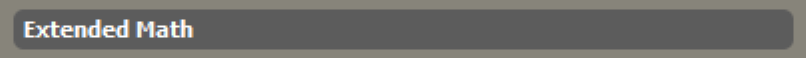----- Constants -----
NaN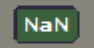Returns a constant NaN which denotes not a number.

Infinity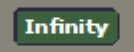Returns a constant which denotes the value of infinity.

Root 2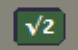Returns the value of the square root of 2.

Phi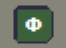Returns Phi (the golden ratio) which is equal to (1 + √5)/2 ≈ 1.618

Maximum Bit IntegersReturns the maximum value of an 8, 16, 32, and 64 bit number.

----- Lists -----
Average, Max, Min values in ListReturns a specific number of the value of items in a given list which matches a desired characteristic.
Choices:
- Average
- Max
- Min

Intersection, Union and Subtraction of ListsReturns a list with a desired modification given 2 lists.
Choices:
- Intersection: Returns a list of items found in both lists.
- Union: Returns a unique set of items found in all lists.
- Subtraction: Returns the first list with all values from the second list removed in the first.

Subset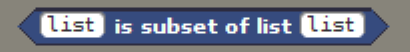Returns true if every item is list A is also found in list B.

Summation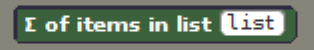Returns the sum of all values in a given list.

Identity Matrix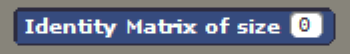Returns a matrix square of a given size with 1's along the diagonal

----- Math -----
Is Number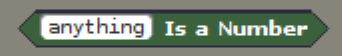Returns true if a given value is a number.

Is Even/OddReturns whether or not a number is even or odd.

+/-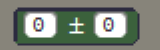Returns a list of size 2 where the value of A + B is at index 0 and A - B is at index 1.

Inverse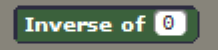Returns the inverse 1/N of a number.

Divides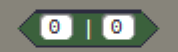Returns true if the first number evenly divides the second.

Modular Arithmetic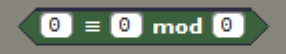Returns true if the remainder of each of the first two numbers divided by the last are the same.

Cube Root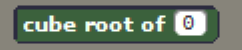Returns the cube root of a number.

N-th Root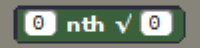Returns the N-th root of a number

Log with Base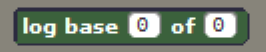Returns the logarithm of a number with any base.

Factorial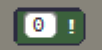Returns the factorial of a number. ie. (N)(N-1)(N-2)...(1)

Pick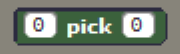Returns the factorial of a number to a given step. ie. (N)(N-1)(N-2)...(N-(k-1))

Choose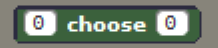Returns ((N)!/(N-k)!)/(k)!

sinh, cosh, tanh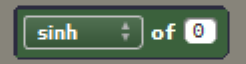Returns sinh, cosh, or tanh.
~ sinh = (1/2) (-(1/e^N) + e^N)
~ cosh = (1/2) ((1/e^N) + e^N)
~ tanh = sinh(N)/cosh(N)

Implies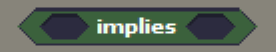Returns false if the premise is true, but the outcome is false otherwise returns true.

Heaven Side Step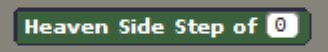Returns 0 if the number given is 0, 1 if the number given is larger than 0, and -1 if the number given is less than 0.

----- Positional Space -----
Distance Between ActorsReturns the distance between 2 actors in the scene.

----- Geometry -----
CircleReturns the area or circumference of a circle with radius R.

RectangleReturns the area or perimeter of a rectangle with length L and width W.

Last Updated April 7, 2020 v1.0
-------------------------------------------------------------------------------------------------------------------------

Contact Me: thejoblesscoder@gmail.com

Extension is Open Sourced and Licensed under MIT open source standards.
Games: Arrow Mania, Breakout/Outbreak, Water Drop

Nice work!

#### yoplalala

Nice extension  !  I like some of the functions.

So I have multiple comments to improve the code and a few ideas for some functions

Code: [Select]
`public static function PlusMinus(_A:Float,_B:Float):Array<Dynamic> { var plusminus:Array<Dynamic> = new Array<Dynamic>(); plusminus.push(_A + _B); plusminus.push(_A - _B); return plusminus; }`Use Array<Float> instead of Array<Dynamic> .  Try to use dynamic only if you need to.

Same here

Code: [Select]
`public static function IdentityMatrix(n:Int):Array<Dynamic> { n = Math.floor(n); var list = new Array<Dynamic>(); for(i in 0...n) { list.push(new Array<Dynamic>());`You can use Array<Array<Bool>>     and Array<Bool>

You have multiple  functions

Code: [Select]
`public static function NaN():Float { return Math.NaN; }`
That you can put directly in the blocks.xml.  Also I think they are many functions you can inline to improve the performances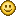https://haxe.org/manual/class-field-inline.html

The ideas will be posted in an other post.

#### MrD69

Hey thanks for the suggestions I will look into that. As for the Array<Dynamic> returns I believe that is because all arrays used in Stencyl are of the dynamic type and so trying to return an Array of type Float causes errors on compiling.
Games: Arrow Mania, Breakout/Outbreak, Water Drop